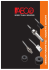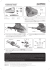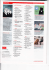# Lecture 13

## Transcription

Lecture 13
```Homework (lecture 11):
3, 5, 9, 13, 21, 25, 29, 31, 40, 45, 49, 51, 57, 62

6
6
ˆ
ˆj
v

(
2
.
0

10
m
/
s
)
i

(
3
.
0

10
m
/
s
)
3. An electron that has velocity:

moves through the uniform magnetic field B  (0.030T )iˆ  (0.15T ) ˆj
(a) Find the force on the electron. (b) Repeat your calculation for a
proton having the same velocity.
Recall:
  
c  a b
 c1   a2b3  a3b2 

  
 c2    a3b1  a1b3 
c  a b  a b 
2 1
 3  1 2
(a) The force acts on the electron:

 
F  qv  B

F  q(v x B y  v y Bx )kˆ

F  (1.6 1019 )[ 2 106  (0.15)  (3 106  0.03)]kˆ

14 ˆ
F  6.24 10
k
(b) The proton has a positive charge:


14
F p  6.24 10
kˆ
So, the force acting on the proton has the same magnitude but
opposite in direction to the force acting on the electron
5. An electron moves through a uniform magnetic field given by
B  Bxiˆ  (3.0Bx ) ˆj . At a particular instant, the electron has

velocity v  (2.0iˆ  4.0 ˆj )m / s and the magnetic force acting on it is
(6.4 10 19 N )kˆ Find Bx.

F  q(v x B y  v y Bx )kˆ  Fz kˆ
Fz
Bx 
q(3v x  v y )
(T )
9. In Fig. 28-33, an electron accelerated from rest through
potential difference V1 = 1.00 kV enters the gap between two
parallel plates having separation d = 20.0 mm and potential
difference V2 = 100 V. The lower plate is at the lower potential.
Neglect fringing and assume that the electron's velocity vector is
perpendicular to the electric field vector between the plates. In
unit-vector notation, what uniform magnetic field allows the
electron to travel in a straight line in the gap? Animation


The electric force: F  qE
e
V2
eV2
E
 Fe 
d
d
Fe

E

B
+

FB
The magnetic field force acts on the electron with an external B:

 
FB  qv  B  FB  evB
1 2
mv  eV1  v 
2
2eV1
m
To travel in a straight line:


Fe  FB
eV2
V2
 evB  B 
d
vd
B
V2
2eV1
d
m
100


3
20 10

19
2 1.6 10
1000
B  2.67 10
9.1110 31
4
(T )

4 ˆ
B  (2.67 10 T )k
13. A strip of copper 150 m thick and 4.5 mm wide is placed in a
uniform magnetic field B of magnitude 0.65 T, with B perpendicular
to the strip. A current i = 23 A is then sent through the strip such
that a Hall potential difference V appears across the width of the
strip. Calculate V. (The number of charge carriers per unit volume
for copper is 8.47 x 1028 electron/m3).
Bi
n
Vle
l: thickness of the strip
V
0.65  23
8.47 10 28 150 10 6 1.6 10 19
V  7.4 10 6 (V )
21. An electron of kinetic energy 1.20 keV circles in a plane
perpendicular to a uniform magnetic field. The orbit radius is 25.0
cm. Find (a) the electron's speed, (b) the magnetic field magnitude,
(c) the circling frequency, and (d) the period of the motion.
(a) The speed:
1 2
K  mv  v 
2
2K
m
(b) The centripetal force here is the magnetic force:
2
v
mv
F  ma  m
 qvB  B 
R
qR
(c) The frequency:
1
v
f  
( Hz )
T 2R
(d) The period:
1
T
( s)
f
25. (a) Find the frequency of revolution of an electron with an
energy of 100 eV in a uniform magnetic field of magnitude 35.0 T.
(b) Calculate the radius of the path of this electron if its velocity
is perpendicular to the magnetic field.
(a) the frequency:
qB
f 
2m
eB
For the electron case: f 
2me
1.6 10 19  35.0 10 6
f 
2  3.14  9.1110 31
5
f  9.8 10 ( Hz )
2 Kme
mv m 2 K / m
r


 0.96(m)
qB
qB
eB
29. An electron follows a helical path in a uniform magnetic field of
magnitude 0.300 T. The pitch of the path is 6.00 m, and the
magnitude of the magnetic force on the electron is 2.00 x 10-15 N.
What is the electron's speed?
v||  v cos  and v  v sin 
FB  qv B
FB
2 10 15
 v 

 4.17 10 4 (m / s)
eB 1.6 10 19  0.3
The period:
2r 2m
p
T

;T 
v
qB
v ||
qBp
eBp
1.6 10 19  0.3  6 10 6
v ||


31
2m 2me
2  3.14  9.1110
v || 5.03 10 4 (m / s)
2
2
4
v  v||  v  6.53 10 (m / s)
31. A particular type of fundamental particle decays by
transforming into an electron e- and a positron e+. Suppose the
decaying particle is at rest in a uniform magnetic field B of
magnitude 3.53 mT and the e- and e+ move away from the decay
point in paths lying in a plane perpendicular to B. How long after
the decay do the e- and e+ collide?
 ray
When the electron and the positron travel
half of the circular path, they will collide:
me
1
9
t T
 5.110 ( s)
2
eB

B
e

e

40. A wire 2.30 m long carries a current of 13.0 A and makes an
angle of 35.00 with a uniform magnetic field of magnitude
B = 1.5 T. Calculate the magnetic force on the wire.

 
FB  iL  B
FB  iLB sin 
FB  13  2.3 1.5  sin 35  25.7( N )
45. A wire 50.0 cm long carries a 0.500 A current in the positive
direction
of an x axis through a magnetic field:

B  (3.0mT ) ˆj  (10.0mT )kˆ . In unit-vector notation, what is the
magnetic force on the wire?

 
FB  iL  B

L  (0.5m)iˆ  0 ˆj  0kˆ

B  0iˆ  (3.0 10 3T ) ˆj  (10 2 T )kˆ

2 ˆ
3 ˆ
 FB  i[( 0.5 10 ) j  (0.5  3.0 10 )k ]

3 ˆ
3 ˆ
FB  (2.5 10 ) j  (0.75 10 )k
49. Figure 28-41 shows a rectangular 20-turn coil of wire, of
dimensions 10 cm by 5.0 cm. It carries a current of 0.10 A and
is hinged along one long side. It is mounted in the xy plane, at
angle  = 300 to the direction of a uniform magnetic field of
magnitude 0.50 T. In unit-vector notation, what is the torque
acting on the coil about the hinge line.

  B
  ( NiA)B sin '
’ is the angle between n and B


 ' 120
  20  0.1 (50 10
4
0
0
)  0.5  sin120  4.33 10
3
Using the right hand rule to determine the torque direction:


n
  (4.33 10
3
N .m) ˆj
( N .m)
51. Figure 28-44 shows a wood cylinder of mass m = 0.250 kg and
length L = 0.100 m, with N = 10.0 turns of wire wrapped around it
longitudinally, so that the plane of the wire coil contains the long
central axis of the cylinder. The cylinder is released on a plane
inclined at an angle  to the horizontal, with the plane of the coil
parallel to the incline plane. If there is a vertical uniform magnetic
field of magnitude 0.500 T, what is the least current i through the
coil that keeps the cylinder from rolling down the plane?

F2

fs

F4

F3 
Fg

F1
• To keep the cylinder from rolling down:
(1) The net force is zero:
mg sin  f s  ma  0
fs: frictional force
AND
(2) The net torque acting on the cylinder
about the cylinder axis must be zero:
   f   B  B sin  f s r  I  0;   NiA
mgr
mgr
mg
 NiAB sin   mg sin r  0  i 


NAB NL2rB 2 NLB
57. A circular coil of 160 turns has a radius of 1.90 cm.
(a) Calculate the current that results in a magnetic dipole moment
of magnitude 2.30 A.m2. (b) Find the maximum magnitude of the
torque that the coil, carrying this current, can experience in a
uniform 35.0 mT magnetic field.
(a) The magnetic dipole moment:
  NiA
i

NA

2.3
2
160  3.14  0.019
 12.7( A)
(b) The torque acts on the coil:
 is maximum if  = 900:
  B sin
 max  B  2.3  35.0 10
3
 0.081( N .m)
62. In Fig. 28-47a, two concentric coils, lying in the same plane,
carry currents in opposite directions. The current in the larger coil
i is fixed. Current i2 in coil 2 can be varied. Figure 28-47b gives
the net magnetic moment of the two-coil system as a function of i2.
If the current in coil 2 is then reversed, what is the magnitude of
the net magnetic moment of the two-coil system when i2 = 7.0 mA?
• i1 and i2 are in opposite directions:
net  1  2
At i2 = 0:
net  1  2 10
5
2
( A.m )
At i2 = 5 mA:
 net  1  N 2i2 A2  2 10
• if i2 is inversed:
5
 N 2 A2  5 10
 N 2 A2  4.0 10 3
3
0
net  1  N 2i2 A2  2 105  4.0 103  7 103  4.8 105 ( A.m2 )
Homework (lecture 12):
4, 7, 12, 16, 18, 22, 35, 38, 43, 46, 49, 50, 57, 62
4. A straight conductor carrying current i = 5.0 A splits into
identical semicircular arcs as shown in Fig. 29-34. What is the
magnetic field at the center C of the resulting circular loop?
 0i
B
4R
We use the right hand rule to determine
the B field direction of the two arcs:
B  Bupper  Blower  0
7. In Fig. 29-36, two circular arcs have radii a = 13.5 cm and b =
10.7 cm, subtend angle  = 74.00, carry current i = 0.411 A, and
share the same center of curvature P. What are the (a) magnitude
and (b) direction (into or out of the page) of the net magnetic field
at P?
We choose the positive direction if B is
directed into the page
The upper arc produces B directed into the
page and the lower arc produces B out of
the page, so:
0i 0i 0i  1 1 
Bnet  Bupper  Blower 


  
4a
4b
4  a b 

7

4 10  0.411 (74   / 180) 
1
1


Bnet 


2
2 
4
10.7 10 
 13.5 10
 Bnet  1.03 107 (T )
the page
and the net field direction is out of
12. In Fig. 29-38, two long straight wires at separation d = 16.0
cm carry currents i1 = 3.61 mA and i2 = 3.00i1 out of the page. (a)
At what point on the x axis shown is the net magnetic field due to
the
currents equal to zero? (b) If the two currents are doubled, is the
point of zero magnetic field shifted toward wire 1, shifted toward
wire 2,or unchanged?
• i1: B1 is up (positive)
• i2: B2 is down (negative)
 0i
B
2d
 0i1
 0 i2
Bnet 

2d1 2d 2
(a)
 0i1  1
3

Bnet 


2  d1 d 2


  0  d 2  3d1

 d1  4cm; d 2  12cm
(b) unchanged
16. In Fig. 29-47, two concentric circular loops of wire carrying
current in the same direction lie in the same plane. Loop L has
radius 1.50 cm and carries 4.00 mA. Loop 2 has radius 2.50 cm
and carries 6.00 mA. Loop 2 is to be rotated about a diameter
while the net magnetic field B set up by the two loops at their
common center is measured. Through what angle must loop 2 be
rotated so that the magnitude of that net field is 100 nT?
The net B field:
 0i
B
2R
B 2  B12  B22  2B1B2 cos(1800   )
 B2  B2  B2 


1
2
  arccos


 2 B1B2
  144
0



Bnet

B1


B2
18. A current is set up in a wire loop consisting of a semi-circle of
straight lengths, all in the same plane. Figure 29-48a shows the
arrangement but is not drawn to scale. The magnitude of the
magnetic field produced at the center of curvature is 47.25 T.
The smaller semicircle is then flipped over (rotated) until the loop is
again entirely in the same plane (Fig. 29-48b). The magnetic field
produced at the (same) center of curvature now has magnitude
15.75 T and its direction is reversed. What is the radius of the
smaller semicircle?
 0i
For a semi-circle: B 
4R
 0i  0i  0i  1 1 
Figure a: Ba 


  
4 R 4r
4 R r
 0i  0i  0i  1 1 
Figure b: Bb 


  
4 R 4r
4 R r
R  r Ba
47.25
R


 3  r   2.25(cm)
r  R Bb
15.75
2
22. Figure 29-42a shows, in cross section, two long, parallel wires
carrying current and separated by distance L. The ratio i1/i2 of
their currents is 4.00; the directions of the currents are not
indicated. Figure 29-42b shows the y component By of their net
magnetic field along the x axis to the right of wire 2. (a) At what
value of x > 0 is By maximum? (b) If i2 = 3 mA, what is the value
of that maximum? What is the direction (into or out of the page)
of (c) i1 and (d) i2?
• B = 0 at x = 10 cm,
so i1 and i2 are in opposite
directions:
 0i1
 0 i2
 0 i2  4
1
Bnet 


 

2 ( L  x)
2x
2  L  x x 
• B is maximum when x matches the following equation:
'
1
 4
f ' ( x)  
  0
 L x x
 3x 2  2Lx  L2  0
 x  L; x  L / 3(di scarded as x  0)
Now, we need to calculate L. At x = 10 cm, By = 0, so:
3x  L  0  L  3x  30(cm)
(b) if i2 = 3 mA:
 0 i2  4
1   0 i2
Bnet,max 
 

2  L  L L 
2L
4 10 7  3 10 3
Bnet,max 
 2 10 9 (T )
2  0.3
(c), (d): for x > 10 cm, Bnet > 0, and B1 > B2: so B1 > 0 and
B2 < 0, thus i1 is directed out and i2 into the page
35. Figure 29-55 shows wire 1 in cross section; the wire is long and
straight, carries a current of 4.00 mA out of the page, and is at
distance d1 = 2.40 cm from a surface. Wire 2, which is parallel to
wire 1 and also long, is at horizontal distance d2 = 5.00 cm from
wire 1 and carries a current of 6.80 mA into the page. What is the
x component of the magnetic force per unit length on wire 2 due to
wire 1?

0 Li1i2
F1 
2d
0 Li1i2
F1, x  F1 cos  
cos 
2d
F1, x
0 Li1i2 d 2
0i1i2 d 2
F1, x 


L
2d 2
2 d12  d 22

B1
d



F1

• At x = 4 cm, F2x = 0, so i1 is
in the same direction as i3.
• When i3 approaches i2,
F2 is positive and dominated
by the force from i3, so i2 is
out of the page.

B21

B23
F2x/L2(N/m)
38. Figure 29-57a shows, in cross section, three current carrying
wires that are long, straight, and parallel to one another. Wires 1
and 2 are fixed in place on an x axis, with separation d. Wire 1
has a current of 0.750 A, but the direction of the current is not
given. Wire 3, with a current of 0.250 A out of the page, can be
moved along the x axis to the right of wire 2. As wire 3 is moved,
the magnitude of the net magnetic force F2 on wire 2 due to the
currents in wires 1 and 3 changes. The x component of that force
is F2, and the value per unit length of wire 2 is F2x/L2. Figure 2957b gives F2x/L2 versus the position x of wire 3. The plot has an
asymptote F2x/L2 = -0.627 N/m as x  . What are the (a) size
and (b) direction (into or out of the page) of the current in wire 2?
At x = 4 cm:
F2,net
L2
0i2i3 0i1i2


0
2x
2d

F21
i1
0.75
d  x
4  12(cm)
i3
0.25
When x  :
F2,net
L2
F21 0i1i2

6


 0.627 10
L2
2d
 i2  0.5( A)

B21

B23

F23
43. Figure 29-62 shows a cross section across a diameter of a long
cylindrical conductor of radius a = 2.00 cm carrying uniform current
170 A. What is the magnitude of the current's magnetic field at
radial distance (a) 0, (b) 1.00 cm, (c) 2.00 cm (wire's surface), and
(d) 4.00 cm?
The magnetic field is inside a wire with current:
  0i 
r
B  
2
 2R 
(a) B = 0;
7 170
  0i 
4


10
2 r
(b) B  
r 
r

8
.
5

10

2
2 4 10 4
 2a 
B  8.5 10
(c)
(d)
2
r  8.5 10
2
1.0 10
B  17.0 10
 0i
4
B
 B  8.5 10 (T )
2r
4
(T )
2
 8.5 10
4
(T )
 0i
B
2r
0.5
  0i 
r
B  
2
 2R 
46. Eight wires cut the page perpendicularly at the points shown in
the figure below. A wire labeled with the integer k (k = 1, 2,…,8)
carries the current ki, where i = 6.0 mA. For those wires with odd
k, the current is out of the page; for those with even k, it is into

the page. Evaluate
B.ds along the closed path in the direction
shown.

• Using Ampere’s law:

 
Bds  0ienc  0 (i1  i3 i 6 i7 )
• Using the curled-straight right hand rule:
i1, i3, i7 are positive and i6 is negative
 
 Bds 0 (i  3i  6i  7i)  5i0
 
3
7
8
 Bds 5  6.0 10  4 10  3.77 10 (T .m)
49. A toroid having a square cross section, 5.00 cm on a side, and
an inner radius of 15.0 cm has 500 turns and carries a current of
0.800 A. (It is made up of a square solenoid instead of a round one
as in Fig. 29-17 -bent into a doughnut shape.) What is the
magnetic field inside the toroid at (a) the inner radius and (b) the
 0iN 1
B
2 r
(a) at the inner radius, r = 15 cm:
B

7
4 10  0.8  500
2
1

4
 5.33 10 (T )
0.15
(b) at the outer radius, r = 15 + 5 = 20 cm:
4 10 7  0.8  500 1
B
 4.0 10 4 (T )
2
0.20
50. A solenoid that is 95.0 cm long has a radius of 2.00 cm and a
winding of 1500 turns; it carries a current of 3.60 A. Calculate the
magnitude of the magnetic field inside the solenoid.
The magnetic field inside a solenoid is computed by:
B  0in
where n is the number of turns per unit length
So:
N 1500
n

 1579 ( turns/m)
L
0.95
B  4 10
7
 3.6 1579  7.14 10
3
(T )
57. A student makes a short electromagnet by winding 300 turns of
wire around a wooden cylinder of diameter d = 5.0 cm. The coil is
connected to a battery producing a current of 4.0 A in the wire.
(a) What is the magnitude of the magnetic dipole moment of this
device? (b) At what axial distance z >> d will the magnetic field
have the magnitude 5.0 T (approximately one-tenth that of
Earth's magnetic field)?
(a) The magnetic dipole moment:
  NiA
2
)  2.36(A.m )
0 NiA  0 


0
B( z ) 

z 3
3
3
2B
2z
2z
  300  4.0   (2.5 10
(b) if z >> d:
2 2

7
4


10

2
.
36
z3
 0.46(m)
6
2  5 10
62. In Fig. 29-66, current i = 56.2 mA is set up in a loop having
two radial lengths and two semicircles of radii a = 5.72 cm and b =
8.57 cm with a common center P. What are the (a) magnitude and
(b) direction (into or out of the page) of the magnetic field at P
and the (c) magnitude and (d) direction of the loop's magnetic dipole
moment?
(a) The B field due to a circular arc at its
center:
 0i
B
For the two semicirles:
4R
0i 0i 0i  1 1 
B  Ba  Bb 


  
4a
4b
4 a b
(b) Using the right hand rule, the B direction points into the page
(c) The magnetic dipole moment:
  NiA  iA  i
2
2
 (a  b )

(d)  points into the page (the same direction as n )

2
```

### Formula Sheet for LSU Physics 2113, Third Exam, Fall ’14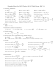### trasportatori e curve conveyors and curves - V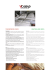### ch29_Magnetic_Induct..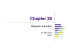### Il Giornale di Vicenza (ITA) - Comitato decennale Luigi Veronelli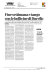### CATALOGO GENERALE ombre:Layout 1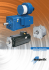### 21/06/2015 Giornale di Brescia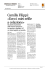### sensori magnetici serie smc